Warning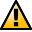This section not yet updated for Inkscape v0.92.

## Shift Tab

The Shift tab allows one to vary the spacing between tiles. With the default parameters, rectangular tiles are arranged so that their Geometric bounding boxes are touching. The following options are available to add or subtract space between the tiles:

• Shift X, Shift Y: Adds (or subtracts) to the tile spacing in units of bounding box width and height. A random factor can also be added.
• Exponent: Changes the exponent factor z so that position of each tile is x (or y) = (1 + shift)z.
• Alternate: The shift alternates between being added and subtracted.
• Cumulate: The previous shift is added to the new shift. For example, if there was a Shift X of 10%, normally the space between subsequent tiles would be 10%, 20%, 30%, and so on. With this option, the shifts become 10%, (10+20)% (10+20+30)%, etc. This is useful when one is also scaling the tiles to keep the tile spacing constant. (See the Scale Tab section for an example.)
• Exclude tile: The tile width or height is excluded in the calculation of tile spacing. This is useful when using the Rotation option to put tiles on a circle. In this case, it is a shortcut for specifying a −100% shift.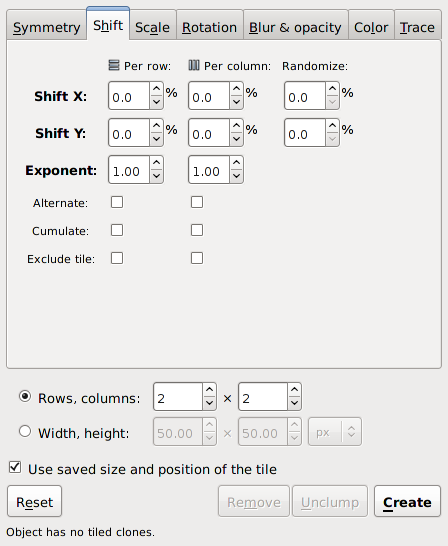The Shift tab of the Tile Clones dialog.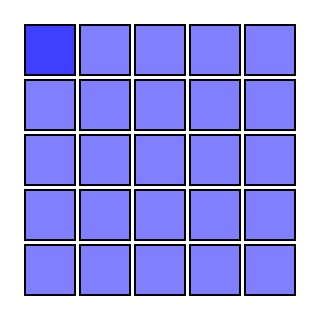A P1 symmetry tiling with a constant shift of 10% (of the bounding box). There is an x shift for each column and a y shift for each row.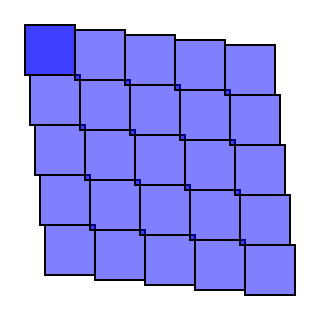A P1 symmetry tiling with a constant shift of 10% (of the bounding box). There is a y shift for each column and an x shift for each row.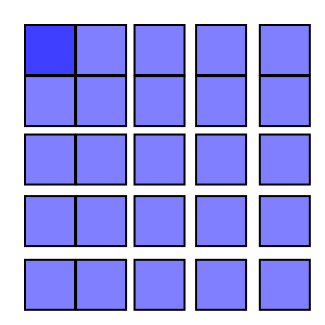A P1 symmetry tiling with an exponential shift of 1.1 (2% shift in x and y).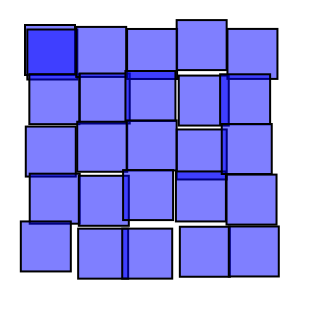A P1 symmetry tiling with a random shift of 10% (of the bounding box) in both x and y.

Question: What is the symmetry of closely packed hexagons? The answer is P1 as can be seen below. One can use this fact to trivially generate the board for the game Hex invented independently by the mathematicians Piet Hein and John Nash.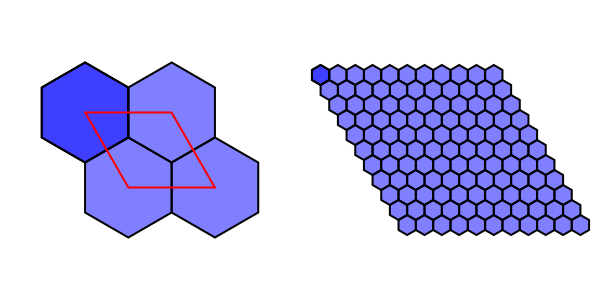Closely packed hexagons have a P1 symmetry tiling as shown on the left. On the right is the board for the game Hex. To generate both tilings, a hexagon was tiled using a shift in x of 50% and a shift in y of −25% per row.